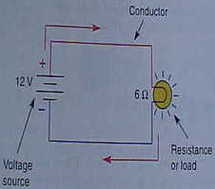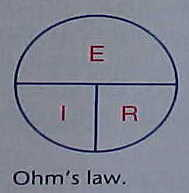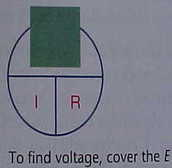# How Ohms law Works

This week I wanted to talk about ohms law since I mentioned it in last week’s post about taking the ASE automotive electrical test. Although you may not use this formula while you are solving automotive electrical problems if you plan on passing the electrical test, understanding the law and its principles can really come in handy.Electrical Circuit

George Ohm is accredited with the term. He was a German mathematics professor and in 1827 wrote a book that included the explanation of how electricity behaved. His thoughts have become the basis for a true understanding of how electricity works.

In his book he states that it takes 1 V of electrical pressure to push one amp of electrical current through a 1 ohm resistor. This equation is the basic law of electricity and is known as ohms law. In a practical example a simple electrical circuit can be connected to a voltage source by conductors better known as wiring.

The resistor could be a lamp and the voltage source could be the battery. The two are connected by wiring. In any electrical circuit resistance (R) current (I) and voltage (E) are related to each other in how the circuit will perform. This can be converted into a mathematical equation. This equation can be applied to the entire circuit or just a section of it.

## How ohms law worksExpert Auto Repair Tip: Take it from someone who knows...Having the online repair manual is a life-saver when it comes to DIY auto repair and maintenance. Whether you are working on a Ford, BMW, Dodge, or something more exotic; we probably have the manual you need.

## Find Your Online Car Repair Manual Today! ->>

Ohms Law

The equation is (E) over (I) times (R). When any two factors of the equation are known the third factor can be determined by using ohms law and the mathematical equation. If you are trying to calculate voltage this would equal the current measured in amps multiplied by the resistance measured in ohms.

If you are looking for the amperage you would then take the voltage and divide by the resistance that is measured in ohms. To find the resistance you can take the voltage and divide by the current that is measured in amps.

As an automotive mechanic it is important to understand ohms law because it truly explains how an increase or decrease in voltage, resistance, or current can affect the operation and performance of an automotive circuit.

## Practical application of ohms lawFinding Voltage

Using the lamp example from above let’s pretend that it is a parking light. If you are trying to calculate how many amps the bulb uses you can measure the resistance of the parking lamp using an automotive meter. You can then take the battery voltage of 12 V and divide it by the resistance to find out exactly how much amp’s the bulb uses.

You may be wondering how this can apply to the real world. What would happen if the resistance in the circuit increased due to a corroded or damaged connection to our parking lamp? If the corroded connection added to ohms of resistance to the parking lamp circuit the amount of amps flowing through the bulb would be decreased.

If you were looking at this bulb it may not seem to be shining as bright as the other bulbs on the vehicle. With this knowledge you now start checking electrical connections in the parking light circuit that has the dim bulb. And you know what you are looking for an increase in resistance causing a decrease in amperage.

I don’t claim in any way to be an automotive electrical instructor. In fact I am just a mechanic that will be taking the ASE recertification for auto electrical systems for the fifth time. On my other website I have about 10 pages that dive into more automotive electrical theories that you may find helpful for solving car electrical problems. If you are looking for more of the most recent articles on this blog this next link will take you to the homepage from this article about ohms law.